## Introduction

Tunable X-ray synchrotron radiation (SR) sources have wide applications in natural sciences, medicine and industry1,2. X-rays for such applications are mostly generated from SR facilities, which are usually bulky, costly and not able to satisfy the increasing demand for researches and applications3. Compact, tunable and flexible light sources are ideal for a broad range of applications and many schemes for such sources are under investigation4,5. Among these the laser wakefield accelerator (LWFA) scheme is highly promising due to its tremendous accelerating gradient6,7,8. Electrons can be longitudinally accelerated to GeV energies over centimeters9. Besides longitudinal acceleration, electrons also undergo transverse betatron oscillations in wakefields, which lead to the emission of electromagnetic radiation in the X-ray range10,11,12. Applications of these radiation sources such as object imaging with high resolution have already been tested13,14,15. Further applications may become possible if some key properties of these radiation sources, such as brightness, spectral features and polarization, can be controlled. Among these the polarization is relatively more difficult to control as it relies on the polarization of the electron betatron motion. However, it is usually transversely isotropic. Although the polarization can be controlled through electron injection selection or driver pulse tilting techniques16,17, the polarization tunability is still not sufficient. Coupling the LWFA with external magnetic undulators would produce tunable radiation. However, both the operation difficulty and the device size increase. External undulators and beam transport systems would enlarge the size of the devices from centimetres to several meters18.

## Results and Discussions

### Laser and electron beam spiral motions inside the plasma channel

We first study the electron motion inside the plasma channel which resembles that in a helical undulator. Stable LWFA usually use plasma channels to guide the laser pulse over many Rayleigh lengths (i.e.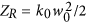, where k0 = 2π/λ0 is the laser wave number, w0 is the focal spot size and λ0 is the laser wavelength). A plasma channel with a transverse density profile of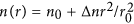can guide the laser for long distances, where r represents the transverse coordinate, n0 is the on-axis electron density, Δn is the channel depth and r0 is the channel width7,22,23. For a matched channel (i.e.,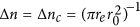and r0 = w0, where re = e2/mec2 is the classical electron radius with e and me the electron charge and mass at rest, respectively and c the light speed in vacuum), the laser spot size remains approximately constant during all propagation. If a laser pulse is off-axially injected into the plasma channel or at some injection angle, the laser centroid will oscillate transversely in a single plane as it propagates forward22. The oscillation period length, given by Λos = 2πZMncn)1/2, where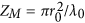, can be controlled by tuning the channel depth22. In addition, when the laser propagation direction is non-collinear with the channel axis, the laser centroid will perform a spiral trajectory around the channel axis as shown in Fig. 1(a). The centroid trajectory can be approximated from the eikonal equations26 (refer to Supplementary Material). In cylindrical coordinates (r, ϕ, z), the laser centroid trajectory is given by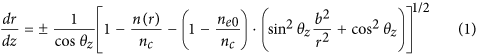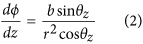with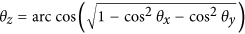and where θx,y,z are the angles between the laser propagation direction and the space coordinate axes x, y and z. Moreover, nc is the critical density of plasma,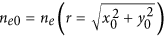is the plasma density at the laser incidence point (x0, y0). The parameter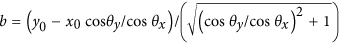is the distance between the projection of the incident laser pulse and the plasma channel centre.

When an ultrashort, ultraintense laser pulse propagates in such a plasma channel, the background electrons are expelled outward due to the pondermotive force of the driver, generating bubble like wakefields27,28. If the laser pulse oscillates transversely, the bubble will follow the laser, forcing electrons inside the bubble to follow the motion of the laser centroid20. The oscillation period and amplitude can be kept constant until near the laser pump depletion22. Thus the plasma acts as a helical undulator and the radiation of the electrons shows typical helical undulator radiation properties, which are dramatically different from those of normal betatron radiation.

We used the fully-relativistic PIC code OSIRIS29 to investigate the self-consistent 3D dynamics of the laser centroid motion and electron acceleration inside the plasma channel when the laser wave vector is not aligned with the channel axis. Detailed simulation parameters can be found in the Methods. The channel axis is set along the z axis. In a typical simulation shown in Fig. 1, the initial parameters of laser incidence are x0 = 1 μm, y0 = 0, θx = 89° and θy = 91°. Typical bubble cross sections at different laser propagation distances are plotted in Fig. 1(b–e). Figure 2(a) shows a typical snapshot of the 3D structure of the laser driven blowout region (yellow), with the externally injected electrons at the back of the bubble (light green) and the laser electric fields (blue-red-orange-dark green). Projections of the laser propagation direction on x-y and x-z planes (black dashed arrows) in Fig. 2(a) and a movie of the laser and wake evolution (refer to the Supplementary Material) indicate the spiral motion. The black solid lines in Fig. 2(b) shows typical trajectory of the laser centroid obtained from theoretical approximations by solving Eqs (1) and (2). The red solid line represents the trajectory from 3D-PIC simulations. The dashed lines on the side planes are the corresponding trajectory projections. The analytical model correctly predicts the wavelength and amplitude of the laser centroid oscillations, both in good agreement with PIC simulations. These results show that the eikonal equations can be used to describe the laser propagation for the typical propagation distances in our investigation. The oscillation wavelength from the PIC simulation is about 700 μm closed to the theoretical approximation Λos = 719.5 μm. In the simulations, because of the laser energy depletion and frequency red shifting as the pulse propagates further, the oscillation wavelength and amplitude become smaller than theoretical predictions. Besides, by comparing laser centroid trajectories and electron beam centroid trajectories with a0 = 2 and a0 = 0.5 (see Supplementary Material), we found relativistic non-linear effects appears when a0 = 2 where trajectories deviate from theoretical evaluations. However, the general trend remains similar and the helical motion of such trajectories is still predicable.

To reduce simulation time and concentrate on the study of the properties of the radiation emitted by the trapped electrons, we consider an external injected scenario, employing a cylindrical electron beam to study the radiation dependance on the intial laser incidence. An illustration of a simulation including ionization injection is given in the Supplementary Material, in order to illustrate the feasibility of our scheme in an all optical setup by only using LWFA. As mentioned before, the trapped and accelerated electron beam experiences spiral motion simutaneously with the acceleration structure following the laser pulse inside the plasma channel. We randomly traced 200 particles from the accelerated electron beam. The centroid position of these electrons (xc, yc, zc) are shown in Fig. 2(b) by the blue solid line. As one can see, the period of the spiral motion of the electrons closely follows the period of the laser centroid oscillations. In addition, the amplitude of the oscillations is slightly higher than the laser centroid oscillations, due to its inertia. Electrons are accelerated to nearly 140 MeV in only 1600 μm. The rms deviation of the average energy increases to about 8 MeV, which results from the nonumiformity of the longitudinal acceleration fields in the helically moving bubble. The continuous acceleration of electrons with a stable accelerating gradient about 80 GeV/m before x = 1600 μm illustrates that electrons propagate near the back of the bubble. After x > 1600 μm, the accelerating gradient reduces rapidly as electrons enter the dephasing region. So the following calculation of electrons trajectories and radiation stops at x = 1600 μm, which corresponds to using a short capillary in practice.

### X-ray radiation from the helical plasma undulator

The spiral motion of the trapped electrons inside the wakefield resembles the electron motion in a traditional helical undulator, which is common in a storage ring of a synchrotron facility for polarized X-ray emission30. We studied the radiation properties of these electrons with the numerical radiation post-processing code VDSR31 (see Methods). This code calculates the radiation distribution according to electrons trajectories obtained from the 3D-PIC OSIRIS simulations. At the beginning of the electron acceleration the normal beam betatron period inside the accelerating bubble (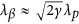) is far smaller than the laser oscillation period (Λos), the radiation properties are more relied on the betatron motion and the emitted photons are low energies which are not the ones we are interested in. In the radiation calculations we cut out these low energy radiation part. Figure 3(a) shows the radiation intensity distribution captured by a virtual radiation detector located in the far-field and centred along the plasma channel axis (see schematic view in Fig. 1(a)). The initial injection parameters of the laser are the same as those in Fig. 1. The far field radiation pattern illustrated in Fig. 3(a) shows a hollow elliptical shape, which is consistent with the electron trajectories whose projection on x-y plane is shown in Fig. 2(b). Key properties of the radiation intensity distribution are described by a fundamental dimensionless radiation parameter, called the strength parameter, given by K = 2πγrosos, where γ is the Lorentz factor of the electrons, ros is their transverse oscillation amplitude and Λos is the spiral motion period. In this case the electrons are rapidly accelerated to high energies (γ ≈ 274). Moreover, the minimum oscillation amplitude is (ros)min ≈ 1.41 μm, the maximum oscillation is (ros)max ≈ 6.84 μm and Λos ≈ 700 μm. These parameters leads to a minimum strength parameter Kmin ≈ 3.5 and a maximum Kmax ≈ 16.8. Both Kmin and Kmax are larger than unity, which means the radiation is in the wiggler regime. As a result, the radiation spectrum is broadband. The X-rays propagate along the emission angles θ ~ K/γ ≈ 0.73° and 3.51°. These angles are larger than the angular width around the emission angle Δ ~ 1/γ ≈ 0.21°, therefore leading to the hollow intensity pattern shown in Fig. 3(a). Besides, more photons are emitted in Fig. 3(a) with azimuth angle ϕ between 135° and 315°. This is because the centroid trajectory of the electron beam ends in such azimuth angle range as well (see Fig. 2(b)). In our scheme, electrons are accelerated and radiate simultaneously. Thus, more intense high frequency radiation is produced when electrons have higher energy. A longer dephasing distance can lead more oscillation times of the electron beam and the impact of electron energy growth on the nonuniformity of the azimuthal angular distribution of the radiation would decrease.

To investigate the spectral properties, we have selected two points with the highest radiation intensities and marked them with black circles. As one can see, the maximum radiation intensity comes from the major axis vertices of the radiation ellipse with emission angle about θ ≈ 3.5°, which corresponds to the positions in the electron trajectory projection plane where the electrons have maximum deviation (ros ≈ (ros)max) and experience maximum transverse acceleration. The photon energy spectra of the marked points are shown in Fig. 3(c,d). The peak position of the spectrum radiated along this direction is about 400 eV, which is about the average value of the on-axis radiation where the peak radiation energy is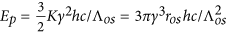with h representing the Plancks constant10. For average electron energy (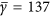) it is Ep = 418.9 eV. In addition, the energy spectra for the point with lowest radiation intensity and integrated over the entire observe region are given in the Supplementary Material.

In order to discuss the polarization properties of the radiated photons, we define the polarization direction degree of a point as P = Ix/It, where Ix is the radiation intensity with photon polarization in the x direction and It is the total radiation intensity. When P = 1 the radiation is linearly polarized in the x direction, while when P = 0 the radiation is linearly polarized in the y direction. The polarization direction degree distribution is shown in Fig. 3(b). The two points marked in Fig. 3(a) were also lined out. During the spiral motion, the directions of the electrons’ transverse velocities keep changing and so does the radiation polarization direction. The polarization direction degree appears to change periodically along the elliptical orbit. When electrons move to the upper right marked position, its velocity gradient component in the x direction is lower than that in the y direction, so P < 0.5 and the radiation intensity in the x direction (corresponding to red line in Fig. 3(c)) is weaker than that in the y direction (blue line in Fig. 3(c)). The opposite situation at the lower left marked position is shown in Fig. 3(d).

In our scheme the intensity and polarization distributions of the radiation can be easily controlled by varying the initial laser incidence parameters, i.e. the initial off-axis position (x0, y0) and the tilted injection angles (θx, θy). In Fig. 4(a) the laser centroid trajectories with different initial parameters are shown. The black line corresponds to the one shown in Fig. 2. The radiation shows hollow elliptical shape. An additional simulation was also performed in order to obtain a hollow and circular intensity profile by using laser injection parameters of x0 = 2.92 μm, y0 = 0 and θx = 90°, θy = 91.5°. The laser performs circular rotation around the channel axis initially. Near laser pump depletion, however, radius of rotation decreases as shown by the red line in Fig. 4(a). Because the emission angle is larger than the angular width of the radiation, the radiation intensity distribution also exhibits a hollow circle pattern, illustrated in Fig. 4(b). The corresponding radiation polarization distribution is shown in Fig. 4(c). As one can see the polarization changes along the azimuthal direction. This kind of distribution is very convenient for many light-polarization-dependent applications.

Quasi linearly polarized X-rays can also be generated by selecting appropriate initial laser incidence. If one fixes the initial incidence position (x0 = 1 μm, y0 = 0) and incidence angle θy = 91°, but only decreases θx from 89° to 88°, the ellipticity decrease of the laser centroid trajectory is evident from the blue line in Fig. 4(a). Correspondingly the electron radiation intensity distribution shows a linear structure (shown in Fig. 4(e)) and a bigger radiation polarization degree P is obtained (see Fig. 4(f)), which means that most of the radiated photons are linearly polarized. Furthermore, in this simulation the oscillation amplitude of the electrons has been increased as one can see in Fig. 4(a). The strength parameter (K) is then increased as well. We find that with these parameters the final peak photon energy moves to 1.5 keV, which shows the tunability of radiation energy. Another way to tune the radiation energy is by varying the channel depth since the strength parameter depends on the laser oscillation period in the parabolic plasma channel. The effects of the channel depth on the radiation parameters has already been studied in detail in refs 22 and 23.

In general, fine tunability of the distribution of the radiation intensity and polarization can be obtained by controlling the combinations of the initial laser incidence parameters. Here we only show the tunability of the elliptical azimuth angle of the radiation pattern by controlling the combined parameter of cos (θx)/cos (θy) with fixed incidence position (x0, y0). The results are shown in Fig. 4(d). The radiation tilt angle can be tuned from 20 to 70 degrees freely. Because of the coincidence of the electron motion and the laser centroid motion, in practice, one can design the radiation properties by solving the eikonal equations of the laser motion first.

## Methods

### Particle-in-Cell simulations

3D-PIC simulations were performed with OSIRIS code. In the simulations, the profile of the normalized laser vector potential is given by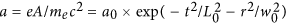with a0 = 2.0 (corresponding to the peak intensity of the polarized laser pulse of I = 8.6 × 1018 W/cm2), L0 = 4.0 T0, w0 = 6.75 λ0 and the laser wavelength λ0 = 0.8 μm, the laser period T0 = 2π/ω0 ≈ 2.67 fs. The plasma density has a parabolic profile with n0 = 0.001 nc, Δn = Δnc, r0 = w0, where nc ≈ 1.7 × 1021 cm−3 is the critical plasma density for the driver pulse. The injected external electron beam has transverse and longitudinal dimensions with size of Lr = 0.5 μm, Lz = 2.0 μm and a charge of Q ≈ 4.5 pC. The energy of the beam is Ee = 7.7 MeV or 5.1 MeV in different simulation cases. The size of the simulation box is 40 × 40 × 45 μm3 corresponding to cell size of 200 × 200 × 1035. We use 4 super-particles per cell and 8 super-particles per cell for the background electrons and external injected electrons, respectively.